# Classs mean

Class size is 30 students, math was not worse than sign 2. Determine the number of pupils who were sign 1 if the class had a 1.4 sign average.

a =  18

### Step-by-step explanation:

a+b= 30
a+2b = 1.4 •30

a+b= 30
a+2•b = 1.4 •30

a+b = 30
a+2b = 42

a = 18
b = 12

Our linear equations calculator calculates it.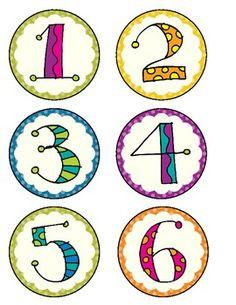Did you find an error or inaccuracy? Feel free to write us. Thank you!Tips to related online calculators
Looking for help with calculating arithmetic mean?
Looking for a statistical calculator?
Do you have a linear equation or system of equations and looking for its solution? Or do you have a quadratic equation?

#### You need to know the following knowledge to solve this word math problem:

We encourage you to watch this tutorial video on this math problem:

## Related math problems and questions:

• Certificate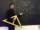There is 31 students in a class. From mathematics was'nt worse mark than 2. Average mark in mathematics was 2. How many students have mark 1 and how many mark 2?
• Exam average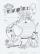The average of marks that have on the certificates students of 8A class in mathematics is exactly 2.45. If we did not add 1 and 3 of students Michael and Alena, who arrived a month ago, it would average exactly 2.5. Determine how many students have class
• Marks at schoolThere are 30 students in the class. Five students in the class had a mark three triple at the end of the math certificate, and the other students had a mark of one or two. The average mark in all students' mathematics in the class at the end of the year w
• Average height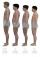In a class are 34 students. The average height of the students is 165 cm. What will be the average height of students in the classroom when two pupils, tall 176 cm and 170 cm, moved from this school/class? The average height in meters of all the students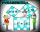Two fifth-graders teams competing in math competitions - in Mathematical Olympiad and Pytagoriade. Of the 33 students competed in at least one of the contest 22 students. Students who competed only in Pytagoriade were twice more than those who just compet
• The bar chartThe bar chart shows the results of the math test. How many% of pupils achieved approximately better results than the average of the class? Diagram: 1-10 pupils, 2-4 pupils, 3-6 pupils, 4-2 pupils, 5-4 pupils. (lowest result are better)
• Math testStudents wrote a math test. The average number of points obtained by them was 64. Another student wrote this test for 80 points. If the teacher added his result to the original ones, the total average of all students would be 65. How many students origina
• Ski class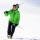Class attend 30 boys and some number of girls. Ski training was attended by 28 boys and all the girls, which was 95% of all students. How many girls attend this class? How many percent is that?
• Average heightThe average height of all pupils is 162 cm. The class teacher's height is 178 cm. The average height of all (teacher and all pupils) is 163 cm. Calculate the number of pupils in the class.
• Jane classWhen asked how many students are in class, Jane said, if we increase the number of students in our class by a hundred % and then add half the number of students, we get 100. How many students are in Jane's class?
• Word problem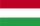348 students were on holiday in Hungary. 133 bought pizza, chips 28 pupils, 25 pupils bought soda, butter 26 pupils, 15 pupils fruits, vegetables 29 students. How much pay for all the food, if every meal cost 5.3 euros?
• In the classroomThere are 30 boys and a few girls in the class. In the six months, 28 boys and all girls benefited, which was 95% of all pupils. How many pupils are there in the classroom?
• Soccer balls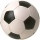Pupils in one class want to buy two soccer balls together. If each of them brings 12.50 euros, they will miss 100 euros; if each brings 16 euros, they will remain 12 euros. How many students are in the class?
• Holidays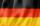Of the 35 students of the class were 7 on holiday in Germany and just as much in Italy. 5 students visited Austria. In none of these countries was 21 students, all three visited by one student. In Italy and Austria were 2 students and in Austria and Germa
• StudentsIf the fifth-grade class left 20% of students and in the seventh grade were added two pupils, in the eighth one pupil, the number has not changed in the ninth grade, but it is now tenth students less than it was in the fifth grade. How many pupils are in
• Christmas or EasterPlease calculate this example by the Venn equation. They asked 73 students whether they like Christmas or Easter. 34 of them like one of the holidays. 39 loves Easter. There are twice as many students who wish both holidays as those who only love Easter.
• Class 8.AThree quarters of class 8.A went skiing. Of those who remained at home one third was ill and the remaining six were on math olympic. How many students have class 8.A?